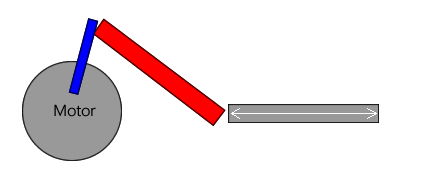# Motor Arm Length Calculator

Below is a picture of what I'm trying to build. This is the motor that I'm using, which has plenty of torque.What I’m trying to figure out is if there is a calculator for what the maximum lengths could be for the blue and red arms of the machine. The grey linear motion will be set at a certain distance away from the motor. All I can find are “piston arm length calculators” which are not exactly what I need for this type of machine I’m thinking? They all seem to focus on too many variables of piston head size, etc.

Does anyone know where I can find a calculator to work out the above lengths?

Thanks for any help.

Logic tells me that the

Logic tells me that the length of the blue arm, from the centre of the motor shaft to the coupling point should be 1/2 the linear distance required, as at the 3 and 9 o’clock positions they define the length of the stroke.  The red arm is whatever length required to produce the required offset to the stroke line.  Remember that the stroke will not be a linear speed however.

the slide lengh (grey with

the slide lengh (grey with arrows) will tell you what is the lengh of the crank (blue)

becarefull:  more “blue” is long, less is the torque for it, you will need to figure how much force you need to push or pull with the slide.  formula of torque: Torque = Load x Radius

example:

motor torque is 10N/10cm (habitually in N/m, in this case 0.1N/m because 10cm is 10 time smaller that the meter (metric so easy))

if arm=10cm => torque=10N (1 KG or 2.2lb)

if arm=20cm=> torque=10N/10cm*2  === 10/20=0.5N (0.5Kg or 1.1lb)

REFERENCE

http://www.societyofrobots.com/mechanics_dynamics.shtml#torqueforce